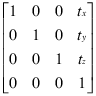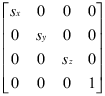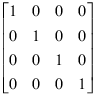## Transforms Supported by hgtransform

### Transforming Objects

The transform object's `Matrix` property applies a transform to all the object’s children in unison. Transforms include rotation, translation, and scaling. Define a transform with a four-by-four transformation matrix.

#### Creating a Transform Matrix

The `makehgtform` function simplifies the construction of matrices to perform rotation, translation, and scaling. For information on creating transform matrices using `makehgtform`, see Nest Transforms for Complex Movements.

### Rotation

Rotation transforms follow the right-hand rule — rotate objects about the x-, y-, or z-axis, with positive angles rotating counterclockwise, while sighting along the respective axis toward the origin. If the angle of rotation is theta, the following matrix defines a rotation of theta about the x-axis.To create a transform matrix for rotation about an arbitrary axis, use the `makehgtform` function.

### Translation

Translation transforms move objects with respect to their current locations. Specify the translation as distances tx, ty, and tz in data space units. The following matrix shows the location of these elements in the transform matrix.### Scaling

Scaling transforms change the sizes of objects. Specify scale factors sx, sy, and sz and construct the following matrix.You cannot use scale factors less than or equal to zero.

### The Default Transform

The default transform is the identity matrix, which you can create with the `eye` function. Here is the identity matrix.### Disallowed Transforms: Perspective

Perspective transforms change the distance at which you view an object. The following matrix is an example of a perspective transform matrix, which MATLAB® graphics does not allow.

`$\left[\begin{array}{cccc}1& 0& 0& 0\\ 0& 1& 0& 0\\ 0& 0& 1& 0\\ 0& 0& {p}_{x}& 0\end{array}\right]$`

In this case, px is the perspective factor.

### Disallowed Transforms: Shear

Shear transforms keep all points along a given line (or plane, in 3-D coordinates) fixed while shifting all other points parallel to the line (plane) proportional to their perpendicular distance from the fixed line (plane). The following matrix is an example of a shear transform matrix, which `hgtransform` does not allow.

`$\left[\begin{array}{cccc}1& {s}_{x}& 0& 0\\ 0& 1& 0& 0\\ 0& 0& 1& 0\\ 0& 0& 0& 1\end{array}\right]$`

In this case, sx is the shear factor and can replace any zero element in an identity matrix.

### Absolute vs. Relative Transforms

Transforms are specified in absolute terms, not relative to the current transform. For example, if you apply a transform that translates the transform object 5 units in the x direction, and then you apply another transform that translates it 4 units in the y direction, the resulting position of the object is 4 units in the y direction from its original position.

If you want transforms to accumulate, you must concatenate the individual transforms into a single matrix. See Combining Transforms into One Matrix.

### Combining Transforms into One Matrix

It is usually more efficient to combine various transform operations into one matrix by concatenating (multiplying) the individual matrices and setting the `Matrix` property to the result. Matrix multiplication is not commutative, so the order in which you multiply the matrices affects the result.

For example, suppose you want to perform an operation that scales, translates, and then rotates. Assuming `R`, `T` and `S` are your individual transform matrices, multiply the matrices as follows:

```C = R*T*S % operations are performed from right to left ```

`S` is the scaling matrix, `T` is the translation matrix, `R` is the rotation matrix, and `C` is the composite of the three operations. Then set the transform object's `Matrix` property to `C`:

```hg = hgtransform('Matrix',C); ```

#### Multiplying the Transform by the Identity Matrix

The following sets of statements are not equivalent. The first set:

```hg.Matrix = C; hg.Matrix = eye(4);```

results in the removal of the transform C. The second set:

```I = eye(4); C = I*R*T*S; hg.Matrix = C;```

applies the transform `C`. Concatenating the identity matrix to other matrices has no effect on the composite matrix.

### Undoing Transform Operations

Because transform operations are specified in absolute terms (not relative to the current transform), you can undo a series of transforms by setting the current transform to the identity matrix. For example:

```hg = hgtransform('Matrix',C); ... hg.Matrix = eye(4);```

returns the objects contained by the transform object, `hg`, to their orientation before applying the transform `C`.

For more information on the identity matrix, see the `eye` function